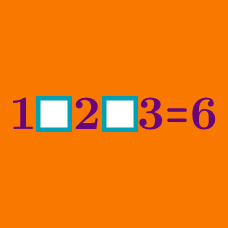Logic

Operator Search Warmup

$1 \square 2 \square 3 \square 4 = 6$

Using only the operators $+$ and $-,$ how should the blanks be filled in to make the equation true?

$4 - 2 \times 3 - 1 =\text{ }?$

If parentheses are inserted into the above statement, which value cannot be made? (The operations cannot be changed otherwise.)

$3 \square 3 \square 3 \square 3 \square 3 = 0$

You want to fill in the squares above to make the equation true, but you are only allowed to use two different types of operators. Which pair of operators will work? (You are not allowed to add parentheses to the above expression.)

Using the numbers 1, 2, 3, 4 and 5 in any order and the operators $+,-,\times, \text{and} \div$ each exactly once, what is the greatest possible value that you can make? You may use any number of parentheses.

$(5 \square 10) - (12 \square 11 \square 2) =\text{ }?$

Using the operators $+, -, \times, \text{and} \div$ to fill in the blanks, what is the maximum possible value of this expression? No parentheses may be added.

×